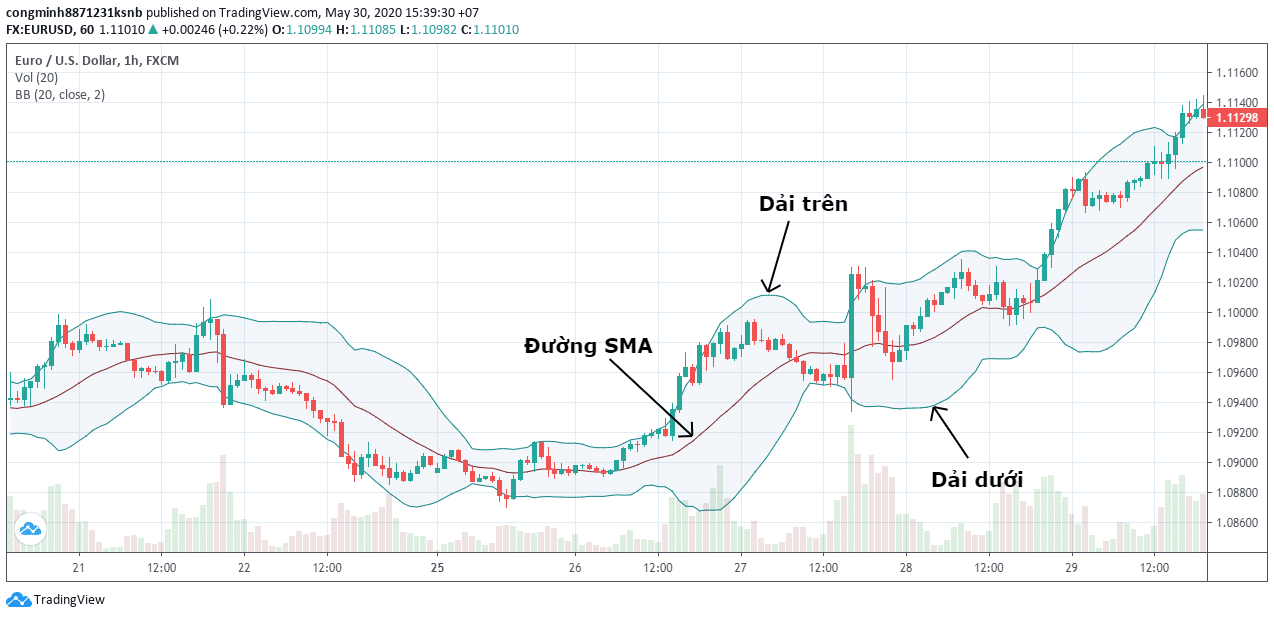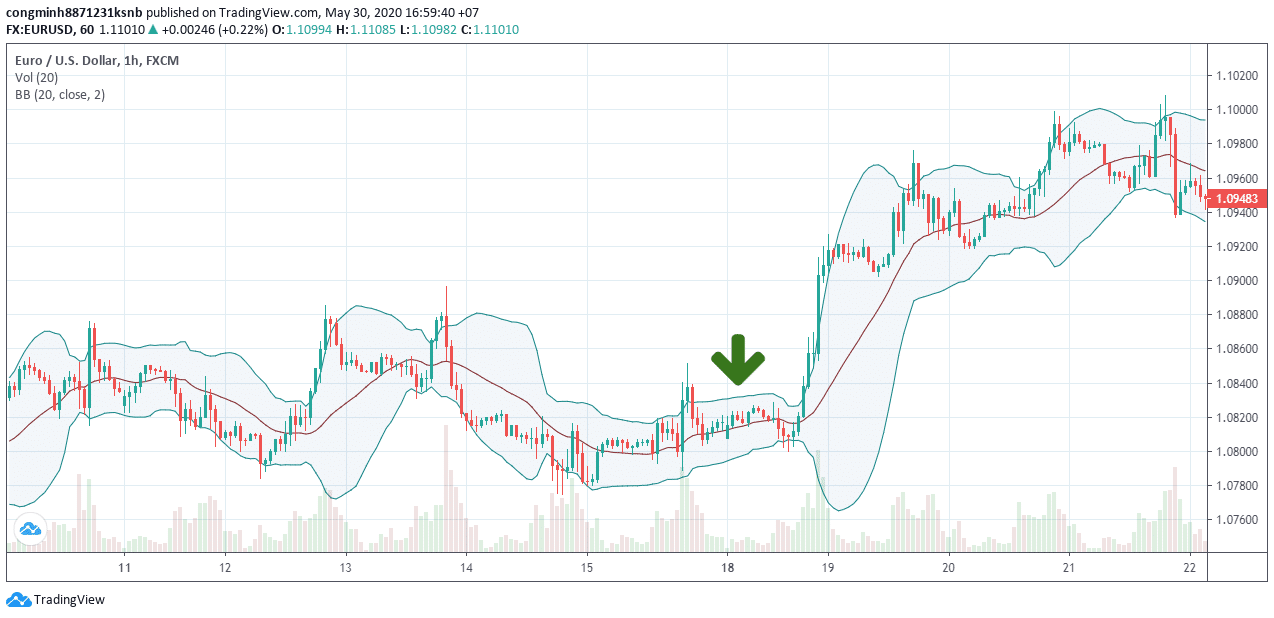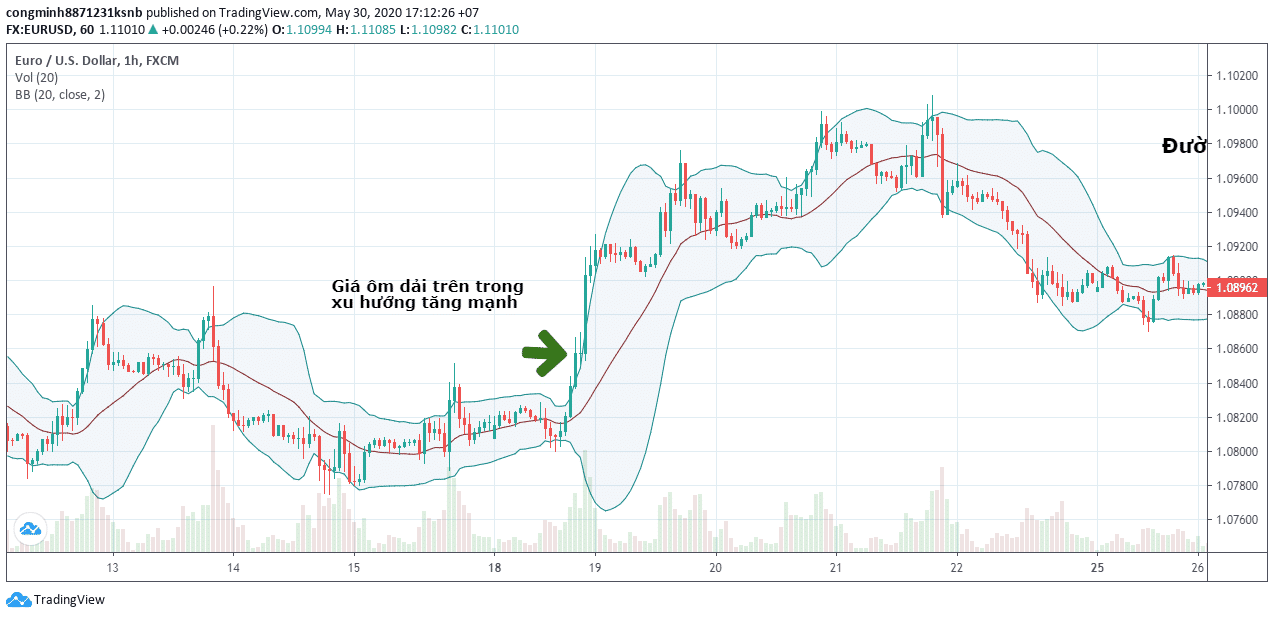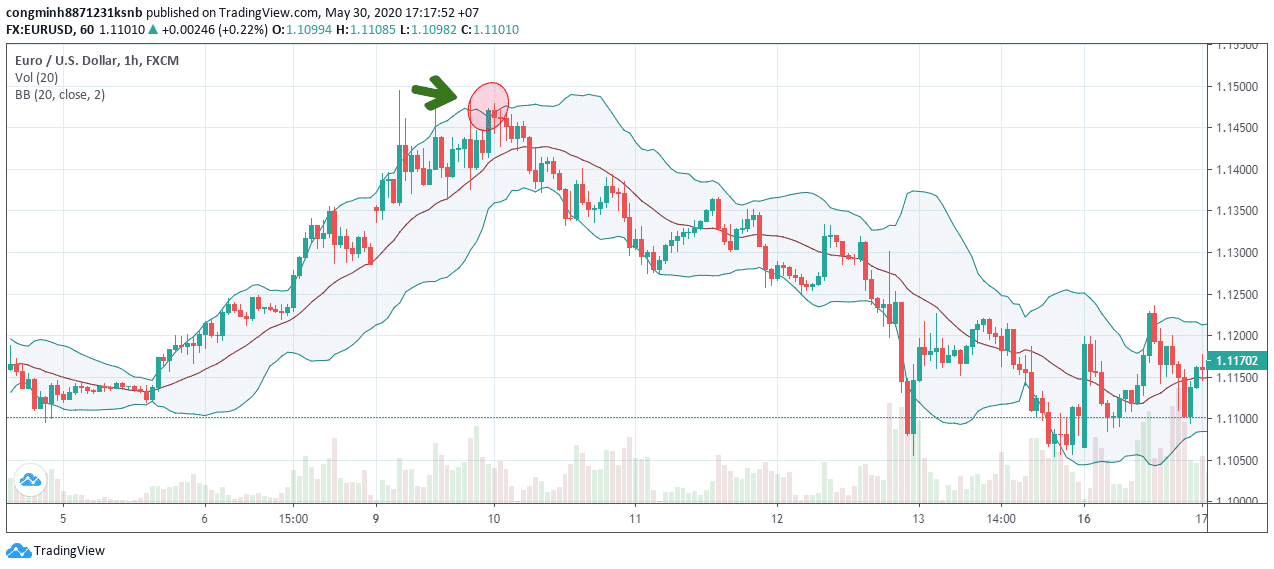# What is a Bollinger band? Practice using Bollinger Bands

0
1215

## What is a Bollinger band?

Bollinger band is an indicator in technical analysis used to measure volatility or signal overbought or oversold of a market.

The bollinger band was created by John Bollinger in the early 1980s. It is widely used by analysts. Based on this, we can tell whether the market is moving or not.

Bollinger bands are applied on a price chart and they represent the standard deviation of a price against Moving averages (MA) its. The distance between the MA line and its Bollinger bands is determined by the degree of price volatility: The faster the price changes, the farther the bands are from the moving average.

## The elements that make up the Bollinger band

There are 3 factors that make up the Bollinger Bands: Mid range, strip on, lower band.### Mid range

The middle of the indicator is a simple moving average (SMA). Current software or charts, most of them show a default of 20 stages. This is also the advice of experienced analysts to use the 20-period MA.

However, after mastering the Bollinger band, you can switch to MA at different stages.

Do not use a moving average with few periods. Because the number of stages in the calculation process is small, it will create a large degree of volatility. This results in less accurate data.

### Upper band and lower band

The upper and lower bands, in order, represent the two standard deviations above and below the moving average.

Standard deviations are defined as quantities used to represent price dispersion around their average:

• A standard deviation: Covers about 68% of price movements that occur.
• Two standard deviations: Covers up to 95% of the price values ​​that appear. This means that if the index is based on the calculation of two standard deviations, 2% of the prices will fluctuate within its ranges.

### Bollinger band calculation formula

Thus it can be seen that the bollinger band uses two parameters: period (period) and standard deviation. The values ​​to shape the standard formula are: period is 2 and standard deviation is 20.

From there the following calculation:

• Mid range: Simple moving average 20-day SMA.
• Upper band: SMA line + 2 x standard deviation.
• Lower band: SMA - 2 x standard deviation.

This is a standard setting and is recommended. But you can still customize it.

For example: Follow the same calculation process but apply to short-term, medium-term and long-term analysis. The parameters can be set as follows:

• Short-term: Use a 10-day SMA, standard deviation 1.5.
• Medium term: Use 20-day SMA, standard deviation 2.
• Long-term: Use a 50-day SMA, standard deviation 2.5.

## How Bollinger band works

- When the sideways price increases for a while, it increases the possibility that the price will increase sharply in either direction. This could initiate a trend. Be wary of a new boom. Described as chart below.- Price tends to bounce within two bands. It touches one strip then touches over another. You can use these changes to help determine the take profit level. Simply put, if the price bounces off the lower band and then crosses the SMA, the upper band will become a profit target if the price gets close.

Price can exceed or hug a band for extended periods during strong trends. In terms of divergences with a momentum oscillator, you might want to do research on whether to take profit or wait for more to increase profits. For example below:- Price moves out of the bands, continuing a strong trend. However, if the price moves immediately back inside the bollinger band, the above trend will be disqualified.Summary of the above issues when it comes to each case. The upper and lower bands are respectively played resistance and support.

However, do not therefore use the concept of resistance support to trade. Just like the other indicators you should combine them with RSI, MACD, ... let the Bollinger band do its best.

## Use Bollinger band and Keltner channel

Keltner Channels is a volatility index introduced by a trader named Chester Keltner in the 1960 book. A later revised version was developed by Linda Raschke in the 1980s.

Different from Bollinger band, this indicator uses exponential moving average (EMA) and actual average moving range (ATR) to represent channel width. It in turn replaces the use of SMA and standard deviations.

Keltner helps to identify overbought and oversold versus the moving average. Especially when the trend doesn't change. Keltner Channels are better able to predict trend reversal points than Bollinger band.

The formula for Keltner Channel also applies to 3 strips only. Common settings include a 20-day EMA and a 10-day ATR.

• Upper band: EMA (20) + 2 x ATR (10).
• Mid range: The exponential moving average 20-day EMA.
• Lower band: EMA (20) + 2 x ATR (10).

In terms of popularity, Bollinger band is still better, guys, but it also has many advantages compared to Keltner Channels. However, if combined that use it is nothing more wonderful. Not only giving good signals but also improving many aspects.

## Summary

So, one more indicator Blogtienao brought to you. Not much, but enough for brothers to enter the market already. First understand these basics first then improve them through practice. At that time, self-improvement will improve profits and minimize losses.

This website uses Akismet to limit spam. Find out how your comments are approved.